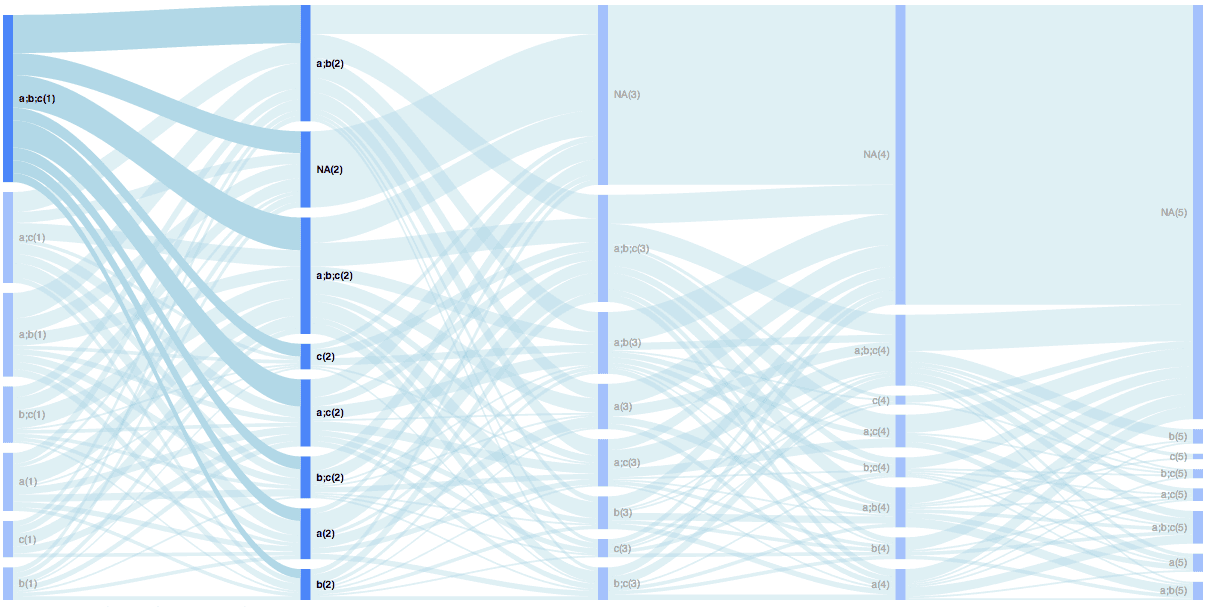Back to Home

Sequence of shopping carts analysis with R – Sankey diagram

We studied how we can visualize the structure of a shopping cart in the previous post. Although you can find plenty of materials on how to analyze combinations of products in the shopping cart (e.g. via association rules), there is a lack of sources on how to analyze the sequences of shopping carts.

This post is an attempt to make up for this lack of sources.

The sequential analysis of the shopping carts can bring you useful knowledge of patterns of customer’s behavior. You can discover dependencies between product sets. For example, the client bought product A and B in the first cart and product A in both the second and third cart. Probably, he wasn’t satisfied with product B (its price, quality, etc.) or you can discover that after “A, B, C” carts clients purchased product D very often. It can give you the opportunity to recommend this product to clients who didn’t purchase D after an “A, B, C” cart.

As I’m a big fan of visualization I will recommend an interesting chart for this analysis: Sankey diagram. So, let’s start!

After we load the necessary libraries with the following code,

```
library(dplyr)
library(reshape2)

```

we will simulate an example of the dataset. Suppose we sell 3 products (or product categories), A, B and C, and each product can be sold with a different probability. Also, a client can purchase any combinations of products. Let’s do this with the following code:

```
# creating an example of orders
set.seed(15)
df <- data.frame(orderId=c(1:1000),
clientId=sample(c(1:300), 1000, replace=TRUE),
prod1=sample(c('NULL','a'), 1000, replace=TRUE, prob=c(0.15, 0.5)),
prod2=sample(c('NULL','b'), 1000, replace=TRUE, prob=c(0.15, 0.3)),
prod3=sample(c('NULL','c'), 1000, replace=TRUE, prob=c(0.15, 0.2)))

# combining products
df\$cart <- paste(df\$prod1, df\$prod2, df\$prod3, sep=';')
df\$cart <- gsub('NULL;|;NULL', '', df\$cart)
df <- df[df\$cart!='NULL', ]

df <- df %>%
select(orderId, clientId, cart) %>%
arrange(clientId, orderId, cart)

```

We generated 1000 orders from 300 clients and our dataset looks like this:

```

```
``` ##    orderId clientId  cart
## 1     451        1  a;b;c
## 2     217        2    a;b
## 3     261        2    a;b
## 4     577        2    a;b
## 5     902        2      c
## 6     199        3  a;b;c```

After this, we need to arrange orders from each client with the following code. Note: we assume that the order/cart serial numbers were assigned based on the purchase date. In other cases, you can use purchase date for identifying the sequence.

```
orders <- df %>%
group_by(clientId) %>%
mutate(n.ord = paste('ord', c(1:n()), sep='')) %>%
ungroup()

```

The head of the data frame we obtain is:

```

```
``` ##   orderId  clientId  cart  n.ord
## 1     451        1  a;b;c   ord1
## 2     217        2    a;b   ord1
## 3     261        2    a;b   ord2
## 4     577        2    a;b   ord3
## 5     902        2      c   ord4
## 6     199        3  a;b;c   ord1```

The next step is to create a matrix with sequences with the following code:

```
orders <- dcast(orders, clientId ~ n.ord, value.var='cart', fun.aggregate = NULL)

```

The head of the data frame we obtain is:

``` ##   clientId  ord1 ord10 ord11 ord2  ord3 ord4 ord5 ord6 ord7 ord8 ord9
## 1        1 a;b;c  <NA>  <NA> <NA>  <NA> <NA> <NA> <NA> <NA> <NA> <NA>
## 2        2   a;b  <NA>  <NA>  a;b   a;b    c <NA> <NA> <NA> <NA> <NA>
## 3        3 a;b;c  <NA>  <NA>  a;b     a <NA> <NA> <NA> <NA> <NA> <NA>
## 4        4   a;c  <NA>  <NA>    a   a;c  b;c  a;b <NA> <NA> <NA> <NA>
## 5        5 a;b;c  <NA>  <NA>  a;c a;b;c    a <NA> <NA> <NA> <NA> <NA>
## 6        6     a  <NA>  <NA>  b;c     b <NA> <NA> <NA> <NA> <NA> <NA>```

Therefore, we just need to choose a number of carts/orders in the sequence we want to analyze. I will choose 5 carts with the following code:

```
orders <- orders %>%
select(ord1, ord2, ord3, ord4, ord5)

```

Also, if you have a lot of product combinations instead of 7 as in my example, you can limit them with the filter() function (e.g. filter(ord1==’a;b;c’)) for clarity.

And finally we will create a data set for plotting with the following code:

```
orders.plot <- data.frame()

for (i in 2:ncol(orders)) {

ord.cache <- orders %>%
group_by(orders[ , i-1], orders[ , i]) %>%
summarise(n=n()) %>%
ungroup()

colnames(ord.cache)[1:2] <- c('from', 'to')

ord.cache\$from <- paste(ord.cache\$from, '(', i-1, ')', sep='')
ord.cache\$to <- paste(ord.cache\$to, '(', i, ')', sep='')

orders.plot <- rbind(orders.plot, ord.cache)

}

```

Note: I added tags to combinations with their number in the sequence because it is impossible to create a Sankey diagram from A product to A product for example. So, I transformed the sequence A –> A to A(1) –> A(2).

Finally, we will get a great type of visualization with the following code:

```
plot(gvisSankey(orders.plot, from='from', to='to', weight='n',